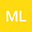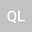A mass supercritical and Sobolev critical fractional Schrödinger system
••Quanqing Li
Honghe University
Author Profile## Abstract

We study the following coupled fractional Schrödinger system: $$\bcs (-\De)^s u=\la_1 u+\mu_1|u|^{p-2}u+\beta r_1|u|^{r_1-2}u|v|^{r_2}\quad &\hbox{in}\;\mathbb{R}^N, \\ (-\De)^s v=\la_2 v+\mu_2|v|^{q-2}v+\beta r_2|u|^{r_1}|v|^{r_2-2}v\quad &\hbox{in}\;\mathbb{R}^N, \\ %\int_{\mathbb{R}^N} u^2=a\quad and\quad \int_{\mathbb{R}^N} v^2=b, \ecs$$ with prescribed mass $\int_{\mathbb{R}^N} u^2=a\quad \hbox{and}\quad \int_{\mathbb{R}^N} v^2=b.$ Here, $a, b>0$ are prescribed, $N>2s, s>\frac{1}{2}$, $2+\frac{4s}{N}0$ sufficiently large, a mountain pass-type normalized solution exists provided $2\leq N\leq 4s$ and \$ 2+\frac{4s}{N}
28 Oct 2021Submitted to Mathematical Methods in the Applied Sciences
29 Oct 2021Submission Checks Completed
29 Oct 2021Assigned to Editor
13 Nov 2021Reviewer(s) Assigned
13 Dec 2021Review(s) Completed, Editorial Evaluation Pending
17 Dec 2021Editorial Decision: Revise Major